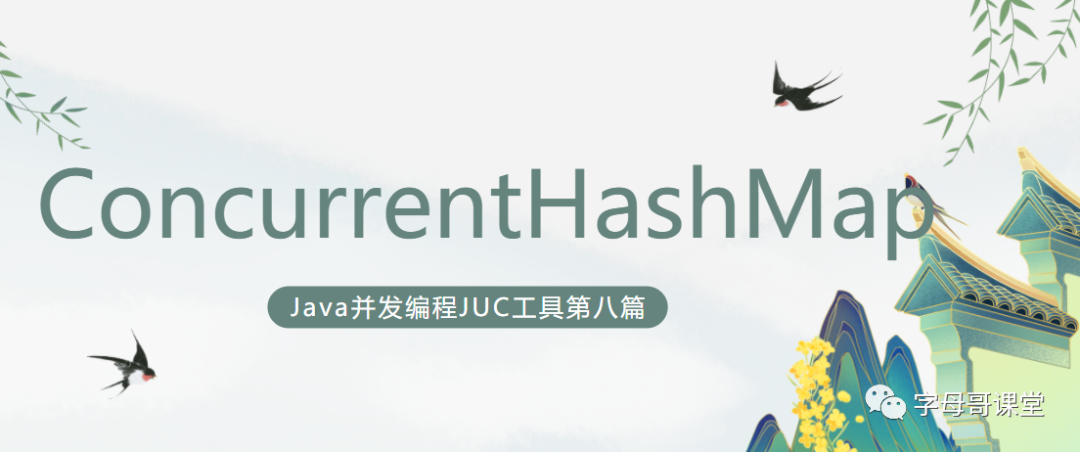# java併發程式設計JUC第八篇：ConcurrentHashMap在之前的文章中已經為大家介紹了java併發程式設計的工具：BlockingQueue介面、ArrayBlockingQueue、DelayQueue、LinkedBlockingQueue、PriorityBlockingQueue、SynchronousQueue、BlockingDeque介面，本文為系列文章第八篇。

`java.util.concurrent.ConcurrentMap`介面是Java集合類框架提供的執行緒安全的map，這意味著多執行緒同時訪問它，不會影響map中每一條資料的一致性。ConcurrentMap介面有兩個實現類ConcurrentHashMap和ConcurrentSkipListMap，經常被使用的是ConcurrentHashMap，我們來重點關注它。

## 1.建立ConcurrentHashMap物件

``// 建立容量為8，負載係數為0.6的ConcurrentHashMapConcurrentHashMap<Key, Value> numbers = new ConcurrentHashMap<>(8, 0.6f);``

• Key - 用於關聯Map中每個元素的唯一標識
• Value - Map中每個元素，可以通過key值獲取value

• capacity容量 - 第一個引數表示這個map的容量是8，也就是說這個物件可以儲存8個鍵值對.
• loadFactor負載因子 - 這個map物件的負載因子是 0.6. 這意味著，每當我們的雜湊表被填滿60%的時候，條目就會被移動到一個新的雜湊表，其容量大小是原來雜湊表的兩倍。

``ConcurrentHashMap<Key, Value> numbers1 = new ConcurrentHashMap<>();``

## 2.ConcurrentHashMap常用方法

### 2.1. 向ConcurrentHashMap插入元素

• `put(K,V)` - 向map中插入key/value 鍵值對資料
• `putAll(map)` - 把另一個map中的所有entries插入到當前的map中
• `putIfAbsent(K,V)` - 向map中插入key/value 鍵值對資料，如果該鍵值對的key在map不存在則插入資料，否則不做操作。
``import java.util.concurrent.ConcurrentHashMap;class Main {    public static void main(String[] args) {        // 建立ConcurrentHashMap 用於儲存偶數        ConcurrentHashMap<String, Integer> evenNumbers = new ConcurrentHashMap<>();        // 使用put()方法插入資料        evenNumbers.put("Two", 2);        evenNumbers.put("Four", 4);        // 使用putIfAbsent()插入資料        evenNumbers.putIfAbsent("Six", 6);        System.out.println("偶數集合ConcurrentHashMap: " + evenNumbers);        //建立ConcurrentHashMap用於儲存整數        ConcurrentHashMap<String, Integer> numbers = new ConcurrentHashMap<>();        numbers.put("One", 1);        // 使用putAll()插入資料        numbers.putAll(evenNumbers);        System.out.println("整數集合ConcurrentHashMap: " + numbers);    }}``

``偶數集合ConcurrentHashMap: {Six=6, Four=4, Two=2}整數集合ConcurrentHashMap: {Six=6, One=1, Four=-4, Two=2}``

### 2.2.批量獲取ConcurrentHashMap 元素

• `entrySet()`- 獲取 map中key/value 鍵值對集合
• `keySet()`- 獲取map中所有的key的集合
• `values()`- 獲取map中所有的value的集合
``import java.util.concurrent.ConcurrentHashMap;class Main {    public static void main(String[] args) {        ConcurrentHashMap<String, Integer> numbers = new ConcurrentHashMap<>();        numbers.put("One", 1);        numbers.put("Two", 2);        numbers.put("Three", 3);        System.out.println("ConcurrentHashMap: " + numbers);        // 獲取 map中key/value 鍵值對集合        System.out.println("Key/Value mappings: " + numbers.entrySet());        // 獲取map中所有的key的集合        System.out.println("Keys: " + numbers.keySet());        // 獲取map中所有的value的集合        System.out.println("Values: " + numbers.values());    }}``

``ConcurrentHashMap: {One=1, Two=2, Three=3}Key/Value mappings: [One=1, Two=2, Three=3]Keys: [One, Two, Three]Values: [1, 2, 3]``

### 2.3. 獲取指定Key元素的value值

• `get()` - 獲取指定key元素的value值，如果key不存在返回null
• `getOrDefault()` - 獲取指定key元素的value值，如果key不存在返回一個指定的預設值
``import java.util.concurrent.ConcurrentHashMap;class Main {    public static void main(String[] args) {        ConcurrentHashMap<String, Integer> numbers = new ConcurrentHashMap<>();        numbers.put("One", 1);        numbers.put("Two", 2);        numbers.put("Three", 3);        System.out.println("ConcurrentHashMap: " + numbers);        // 獲取指定key元素的value值，如果key不存在返回null        int value1 = numbers.get("Three");        System.out.println("Using get(): " + value1);        // 獲取指定key元素的value值，如果key不存在返回一個指定的預設值        int value2 = numbers.getOrDefault("Five", 5);        System.out.println("Using getOrDefault(): " + value2);    }}``

``ConcurrentHashMap: {One=1, Two=2, Three=3}Using get(): 3Using getOrDefault(): 5``

### 2.4.移除ConcurrentHashMap中的元素

• `remove(key)` - 根據指定的key刪除map中的元素，並將該元素返回
• `remove(key, value)` - 只有當map中存在指定的鍵對映到指定的值時，才會從map中刪除條目，並返回一個布林值。返回true表示刪除成功，否則表示map中沒有這個鍵值對。
``import java.util.concurrent.ConcurrentHashMap;class Main {    public static void main(String[] args) {        ConcurrentHashMap<String, Integer> numbers = new ConcurrentHashMap<>();        numbers.put("One", 1);        numbers.put("Two", 2);        numbers.put("Three", 3);        System.out.println("ConcurrentHashMap: " + numbers);        // 根據指定的key刪除map中的元素，並將該元素返回        int value = numbers.remove("Two");        System.out.println("Removed value: " + value);        // 只有當map中存在指定的鍵對映到指定的值時，才會從map中刪除條目，並返回一個布林值。        boolean result = numbers.remove("Three", 3);        System.out.println("Is the entry {Three=3} removed? " + result);        System.out.println("Updated ConcurrentHashMap: " + numbers);    }}``

``ConcurrentHashMap: {One=1, Two=2, Three=3}Removed value: 2Is the entry {Three=3} removed? TrueUpdated ConcurrentHashMap: {One=1}``